# Volume and surface areaPage 1

#### WATCH ALL SLIDES

Slide 1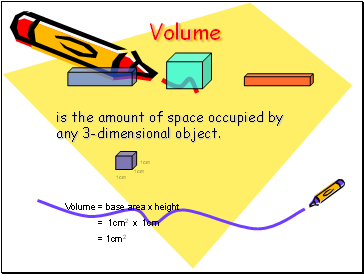## Volume

is the amount of space occupied by any 3-dimensional object.

1cm

1cm

1cm

Volume = base area x height

= 1cm2 x 1cm

= 1cm2

Slide 2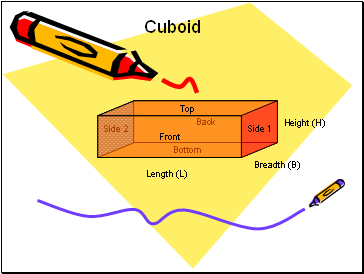## Cuboid

Side 2

Bottom

Back

Top

Side 1

Front

Length (L)

Breadth (B)

Height (H)

Slide 3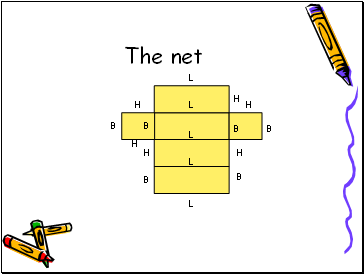The net

L

L

L

L

B

H

H

H

H

L

B

B

B

B

B

H

H

Slide 4H

B

L

H

B

H

L

L

L

H

B

L

H

B

H

L

L

Total surface Area = L x H + B x H + L x H + B x H + L x B + L x B

= 2 LxB + 2BxH + 2LxH

= 2 ( LB + BH + LH )

Total surface Area

Slide 5Slide 6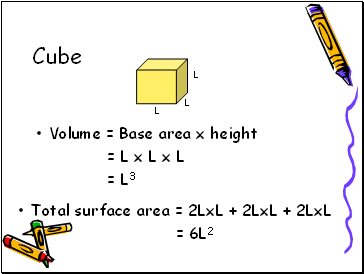## Cube

Volume = Base area x height

= L x L x L

= L3

L

L

L

Total surface area = 2LxL + 2LxL + 2LxL

= 6L2

Slide 7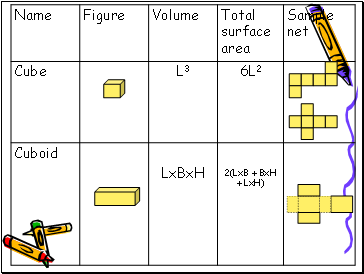2(LxB + BxH + LxH)

LxBxH

Cuboid

6L2

L3

Cube

Sample net

Total surface area

Volume

Figure

Name

Slide 8Show ends

## Last added presentations

© 2010-2021 powerpoint presentations